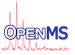OpenMS
ROCCurve Class Reference

ROCCurves show the trade-off in sensitivity and specificity for binary classifiers using different cutoff values. More...

#include <OpenMS/MATH/STATISTICS/ROCCurve.h>

Collaboration diagram for ROCCurve:
[legend]

## Classes

class  simsortdec
predicate for sort() More...

## Public Member Functions

ROCCurve ()
default constructor More...

ROCCurve (const std::vector< std::pair< double, bool >> &pairs)
constructor with value, class pairs More...

virtual ~ROCCurve ()=default
destructor More...

ROCCurve (const ROCCurve &source)
copy constructor More...

ROCCurveoperator= (const ROCCurve &source)
assignment operator More...

void insertPair (double score, bool clas)
insert score, type pair More...

double AUC ()
returns Area Under Curve More...

double rocN (Size N)
returns ROC-N score (e.g. ROC-50). Returns -1 if not enough false positives were found More...

std::vector< std::pair< double, double > > curve (UInt resolution=10)
some points in the ROC Curve More...

double cutoffPos (double fraction=0.95)

double cutoffNeg (double fraction=0.95)

## Private Member Functions

void sort ()
sorts data and caches if sorted More...

void count ()
counts global pos and neg More...

double trapezoidal_area (double x1, double x2, double y1, double y2)

## Private Attributes

std::vector< std::pair< double, bool > > score_clas_pairs_

UInt pos_

UInt neg_

bool sorted_

## Detailed Description

ROCCurves show the trade-off in sensitivity and specificity for binary classifiers using different cutoff values.

[This class is buggy and usage is discouraged!]

## ◆ ROCCurve() [1/3]

 ROCCurve ( )

default constructor

## ◆ ROCCurve() [2/3]

 ROCCurve ( const std::vector< std::pair< double, bool >> & pairs )
explicit

constructor with value, class pairs

## ◆ ~ROCCurve()

 virtual ~ROCCurve ( )
virtualdefault

destructor

## ◆ ROCCurve() [3/3]

 ROCCurve ( const ROCCurve & source )

copy constructor

## ◆ AUC()

 double AUC ( )

returns Area Under Curve

## ◆ count()

 void count ( )
inlineprivate

counts global pos and neg

## ◆ curve()

 std::vector > curve ( UInt resolution = 10 )

some points in the ROC Curve

## ◆ cutoffNeg()

 double cutoffNeg ( double fraction = 0.95 )

returns the score at which you would need to set a cutoff to have fraction positives returns -1 if there are not enough positives

## ◆ cutoffPos()

 double cutoffPos ( double fraction = 0.95 )

returns the score at which you would need to set a cutoff to have fraction positives returns -1 if there are not enough positives

## ◆ insertPair()

 void insertPair ( double score, bool clas )

insert score, type pair

## ◆ operator=()

 ROCCurve& operator= ( const ROCCurve & source )

assignment operator

## ◆ rocN()

 double rocN ( Size N )

returns ROC-N score (e.g. ROC-50). Returns -1 if not enough false positives were found

## ◆ sort()

 void sort ( )
inlineprivate

sorts data and caches if sorted

## ◆ trapezoidal_area()

 double trapezoidal_area ( double x1, double x2, double y1, double y2 )
inlineprivate

calculates area with trapezoidal rule

Parameters
 x1,x2,y1,y2

## ◆ neg_

 UInt neg_
private

## ◆ pos_

 UInt pos_
private

## ◆ score_clas_pairs_

 std::vector > score_clas_pairs_
private

## ◆ sorted_

 bool sorted_
private